Note

This page is a reference documentation. It only explains the function signature, and not how to use it. Please refer to the user guide for the big picture.

# nilearn.image.math_img#

nilearn.image.math_img(formula, **imgs)[source]#

Interpret a numpy based string formula using niimg in named parameters.

New in version 0.2.3.

Parameters:
formula`str`

The mathematical formula to apply to image internal data. It can use numpy imported as ‘np’.

imgsimages (`Nifti1Image` or file names)

Keyword arguments corresponding to the variables in the formula as Nifti images. All input images should have the same geometry (shape, affine).

Returns:
`Nifti1Image`

Result of the formula as a Nifti image. Note that the dimension of the result image can be smaller than the input image. The affine is the same as the input image.

`nilearn.image.mean_img`

To simply compute the mean of multiple images

Notes

This function is the Python equivalent of ImCal in SPM or fslmaths in FSL.

Examples

Let’s load an image using nilearn datasets module:

```>>> from nilearn import datasets
```

Now we can use any numpy function on this image:

```>>> from nilearn.image import math_img
>>> log_img = math_img("np.log(img)", img=anatomical_image)
```

We can also apply mathematical operations on several images:

```>>> result_img = math_img("img1 + img2",
...                       img1=anatomical_image, img2=log_img)
```

## Examples using `nilearn.image.math_img`#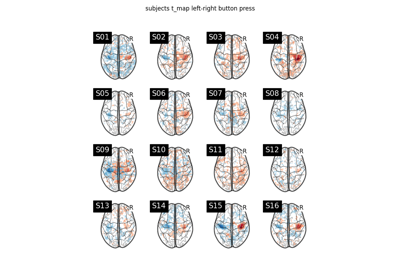Second-level fMRI model: one sample test

Second-level fMRI model: one sample test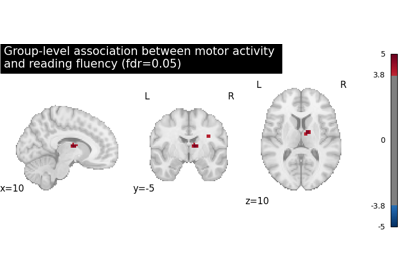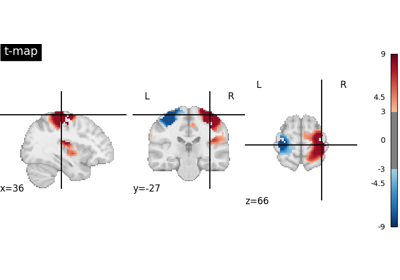Negating an image with math_img

Negating an image with math_img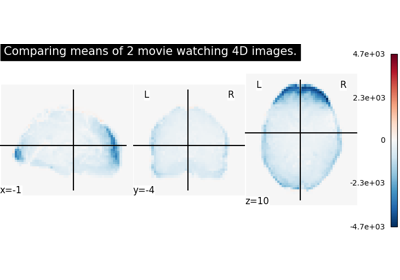Comparing the means of 2 images

Comparing the means of 2 images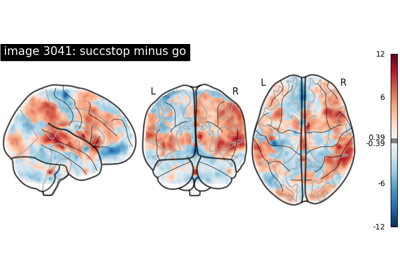NeuroVault meta-analysis of stop-go paradigm studies

NeuroVault meta-analysis of stop-go paradigm studies